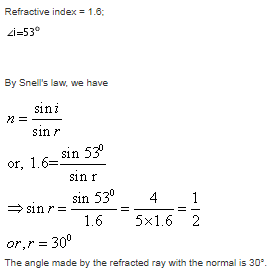# Test: Refraction Of Light

## 15 Questions MCQ Test Additional Documents & Tests for Class 10 | Test: Refraction Of Light

Description
Attempt Test: Refraction Of Light | 15 questions in 15 minutes | Mock test for Class 10 preparation | Free important questions MCQ to study Additional Documents & Tests for Class 10 for Class 10 Exam | Download free PDF with solutions
QUESTION: 1

### A ray of light traveling in air falls on the surface of a transparent glass slab at an angle of 60º with the normal to the surface. Find the angle made by the refracted ray with the normal within the glass slab. Refractive index of the material of the glass slab is 1.5​

Solution:

We know that refractive index of glass slab is 1.5

Here the incidence angle is i=60o

Using snell's law

nairsin(i) = nglasssin(r)

sin60o = 1.5sinr

=> √3/2 = 1.5sinr

=> 1/√3 = sin r

=> r = 35.26o ≈ 35o

QUESTION: 2

### When a beam of light moves from a rarer medium to a denser medium:

Solution:

When light enters from rarer to denser medium its velocity decreases. Hence the ray bends towards normal.

QUESTION: 3

### Fish anticipates the presence of hunter farther due to

Solution:

Light coming from the fish refracts (changes direction) when it hits the surface. A person above the water sees the apparent position of the fish closer to the surface than the real position of the fish.

QUESTION: 4

The bending of a beam of light when it passes obliquely from one medium to another is known as _______.

Solution:

The bouncing back of light is known as reflection of light while refraction of light is the bending light when it pass through one medium to another

QUESTION: 5

You are given kerosene, turpentine and water. In which of these does the light travel fastest ?
The values of refractive index of given substances are:
For kerosene n = 1.44
For turpentine n = 1.47
Water n = 1.33​

Solution:

Optical density of Turpentine is maximum so light travel with minimum speed so,
Speed of light maximum in water then kerosene then minimum in Turpentine.

QUESTION: 6

If the refractive index of two media are equal, then

Solution:

If the refractive index of two medium is same then light wave will travel in a straight line without bending. So we can see that the bending of light will depend upon the refractive index of medium.

QUESTION: 7

Light is refracted when it travels at an angle from water into air because

Solution:

As the light travels from denser medium to lighter medium its speed increases.

QUESTION: 8

According to Snell’s law, the relation between angle of incidence and angle of refraction is

Solution:
QUESTION: 9

The bending of light ray due to change in its speed is

Solution:

Refraction is the bending of a wave when it enters a medium where its speed is different. The refraction of light when it passes from a fast medium to a slow medium bends the light ray toward the normal to the boundary between the two media. The amount of bending depends on the indices of refraction of the two media and is described quantitatively by Snell's Law.

QUESTION: 10

A ray of light travelling in air falls on the surface of a rectangular slab of a plastic material whose refractive index is 1.6. If the incident ray makes an angle of 53º with the normal, find the angle made by the refracted ray with the normal (sin 530 = 4/5)​

Solution:QUESTION: 11

If the speed of light in medium 1 and medium 2 are 2.5 x 102 m/s and 2 x 102 m/s respectively then the refractive index of medium 1 with respect to medium 2 is _______.​

Solution:

Refractive index of medium 1 with respect to medium 2 =  c2 / c1
⇒   2*102 / 2.5*102
⇒     2/2.5

QUESTION: 12

The ratio of sine of angle of incidence to the sine of angle of refraction equals refractive index is called

Solution:
QUESTION: 13

When a ray of light passes from a denser medium to a rarer medium,

Solution:
QUESTION: 14

Light changes its path as the medium changes during the transit. This is because of

Solution:

Its because the velocity of light varies according to the medium,as the speed decreases and increases in different medium,this makes light ray to move towards or away from normal by changing its direction or path in that medium.

QUESTION: 15

A coin is placed at the bottom of a bowl and water is poured into it. The coin appears to be ________ due to _______.

Solution:

A coin placed at the bottom ofa tank appears to be raised when water is poured into it. It happens due to the phenomenon of refraction of light. The point from which the refracted rays appear to come gives the apparent position of the coin.Use Code STAYHOME200 and get INR 200 additional OFF Use Coupon Code